The VARMAX Procedure

Model Diagnostic Checks

Subsections:

Multivariate Model Diagnostic Checks

• Information Criterion After fitting some candidate models to the data, various model selection criteria (normalized by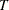) can be used to choose the appropriate model. The following list includes the Akaike information criterion (AIC), the corrected Akaike information criterion (AICC), the final prediction error criterion (FPE), the Hannan-Quinn criterion (HQC), and the Schwarz Bayesian criterion (SBC, also referred to as BIC):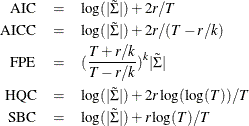where r denotes the number of parameters estimated, k is the number of dependent variables,is the number of observations used to estimate the model, and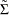is the maximum likelihood estimate of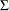. When comparing models, choose the model with the smallest criterion values.

An example of the output was displayed in Figure 35.4.

• Portmanteau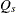statistic The Portmanteaustatistic is used to test whether correlation remains on the model residuals. The null hypothesis is that the residuals are uncorrelated. Let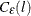be the residual cross-covariance matrices,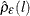be the residual cross-correlation matrices as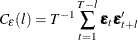and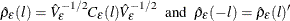where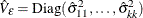and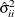are the diagonal elements of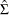. The multivariate portmanteau test defined in Hosking (1980) is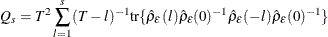The statistichas approximately the chi-square distribution with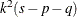degrees of freedom. An example of the output is displayed in Figure 35.7.

Univariate Model Diagnostic Checks

There are various ways to perform diagnostic checks for a univariate model. For details, see the section Testing for Nonlinear Dependence: Heteroscedasticity Tests in Chapter 8: The AUTOREG Procedure. An example of the output is displayed in Figure 35.8 and Figure 35.9.

• Durbin-Watson (DW) statistics: The DW test statistics test for the first order autocorrelation in the residuals.

• Jarque-Bera normality test: This test is helpful in determining whether the model residuals represent a white noise process. This tests the null hypothesis that the residuals have normality.

•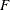tests for autoregressive conditional heteroscedastic (ARCH) disturbances:test statistics test for the heteroscedastic disturbances in the residuals. This tests the null hypothesis that the residuals have equal covariances

•tests for AR disturbance: These test statistics are computed from the residuals of the univariate AR(1), AR(1,2), AR(1,2,3) and AR(1,2,3,4) models to test the null hypothesis that the residuals are uncorrelated.